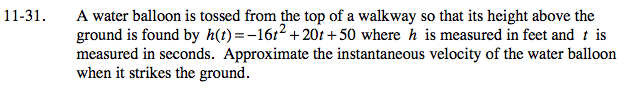### Home > PC > Chapter 11 > Lesson 11.1.2 > Problem11-31

11-31.First determine when the ball hits the ground, or when h(t) = 0.

The ball hits the ground when t = 2.5 seconds.

The velocity is the instantaneous rate of change (IROC). See the Math Notes box in Lesson 9.2.1 for the formula.

$\lim_{h\to 0 }\frac{h(2.5+h)-h(2.5)}{h}$

$\lim_{h\to 0 }\frac{(-16(2.5+h)^2+20(2.5+h)+50)-(-16(2.5)^2+20(2.5)+50)}{h}$